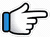# Dil Galti Kar Baitha Hai – Keyboard Notes## Dil Galti Kar Baitha Hai Keyboard Notes

For Dil Galti Kar Baitha Hai Notes as Western or Indian Notations, Click –

Western – CDE FGAB

Indian – srg mpdn

Hindi – सारेग मपधनि

## Keyboard Notes for Dil Galti Kar Baitha Hai – Indian Notation (srg mpdn)

CDE FGAB

सारेग मपधनि

### Part 1

D D   d            n    n R*G*     R*
Yeh_ humne   so_ch_ rakha  tha

R*n~ d          R*~   dn~d        D
Mohabbat   na      karenge    hum
Or
R*n~d          R*~  ndndD    D

DD     d     n      nR*   G*R*
Kisi    ko   dil    nahi   denge

R*n~ d     R*~   dn~d       D
Kisi  pe    na     marenge   hum

Repeat:

D D   d            n    n R*G*     R*
Yeh_ humne   so_ch_ rakha  tha

R*n~ d          R*~   dn~d        D
Mohabbat   na      karenge    hum

DD     d     n      nR*   G*R*
Kisi    ko   dil    nahi   denge

R*n~ d     R*~   dn~d       D
Kisi  pe    na     marenge   hum

### Part 2

DDg*G*g*    R*~ n      dn          R*
Tumhari       mast        aakhon   ne
Or
DDg*G*g*    R*~ R*     dn          R*

Notes are same for next 3 lines:

D  Dg*G*g*     R*~ n     d      nG*
Yeh_ kaisa      haal        kar   dala

D  Dg*G*g*      R*~ n    dn    R*
Ke_ yeh_ dil     cheez     kya   hai

DD          g*G*g*      R*~ n    dn         R*
Maang    lo   toh      jaan      denge   hum

### Part 3

D*D*    D*     M*D*~ M*g*
Palat    ke      ish_       q_  ki

g*G*g*M*      g*    G*R*
Galiyon        se     jaana
Or
G*G*M*~     g*    G*R*

R*g*     G*R*~    nR*g*G*R*ndDdR*
Or
R*g*   G*R*~    nR*g*G*R*ndDR*
R*g*   G*R*~    nR*dDdR*

### Part 4

R*      R*R*     n~  R*G*G*     R*
Dil     galti     kar_ baitha     hai
Or
R*    R*R*     R*nR*G*G*     R*

R*R*     R*   ndn       d     D
Galti    kar  baitha    hai   dil

Repeat:

R*     R*R*   n~    R*G*G*     R*
Dil   galti    kar_ baitha       hai
Or
R*  R*R*   R*nR*G*G*    R*

R*R*    R*     ndn       d      D
Galti   kar    baitha    hai   dil

D*    D*D*   M*d*    D*M*
Dil   galti     kar       baitha

g*G*    M*     g*G*R*
ab       bol      hamara

R*g*    G*R*nD
kya      hoga
Or
d           R*nD

### Part 5

ng*~      G*g*G*R*    ndndD
Bol         hamara       hamara
Or
ng*G*   g*G*R*R*    ndndD
ng*G*   g*G*R*R*    R*nD

ng*~        G*g*G*R*    ndndD
Bol           hamara       hamara

ng*~        G*g*G*R*
Bol           hamara

D*     M*d*D*M*
Oh     Yaara

G*M*   g*G*R*     n      R*dDR*
Bol      hamara    kya   hoga

### Part 6

D*M*g*   g*D*  D*D*
Khamo_shiyan     sunte

D*M* g*      g*M*M*  M*
Kab  tak     rahenge    hum

M*g*G*  G*     M*~M*~    G*R*n
Mauka  hai     hothon         se

nn     g*g*      G*   R*R*
Aaj    kuchh   toh     kaho
Or
nR*  g*g*     G*   R*R*

### Part 7

Notes Similar to Part 6

D*M*     g*~  g*D*   D*D*

D*M*  g*~  g*M*    M*M*
Yeh     zindagi        yaara

M*g*G*G*         M* M* G*R*n
Zara   paas         aa    jao~

nng*g*     G*     R*R*
Sochte     na     raho

### Part 8

Notes Similar to Part 2

D  Dg*G*g*R*          n   dn          R*
Tum_ apna_   lo      ya   thukra   do

Notes are same for next 3 lines:

DDg*         G*g*   R*~ nd   n    G*
Tumhein   haq     faisle      ka   hai

DDg*        G*g*   R*~ nd      n     R*
Humein   kya       puchhte   ho   tum

DDg* G*g*    R*~ n d        n       R*
Humare        hath_mein   kya    hai

### Part 9

Notes Similar to Part 3

D*    D*      D*    M*D*~ M*g*
Ke   hum   toh   kar        chuke

g*G*g*M*    g*    G*R*
Jaana           tumhe
Or
G*G*M*~   g*    G*R*

R*g*   G*~ R*n        R*g*G*R*ndDdR*
Har   saans mein     shamil
Or
R*g*   G*~ R*n       R*g*G*R*ndDR*
R*g*   G*R*~         nR*dDdR*

### Repeat Part 4 and 5

R*      R*R*     n~  R*G*G*     R*
Dil     galti     kar_ baitha     hai
Or
R*    R*R*     R*nR*G*G*     R*

R*R*     R*   ndn       d     D
Galti    kar  baitha    hai   dil

Repeat:

R*     R*R*   n~    R*G*G*     R*
Dil   galti    kar_ baitha       hai
Or
R*  R*R*   R*nR*G*G*    R*

R*R*    R*     ndn       d      D
Galti   kar    baitha    hai   dil

D*    D*D*   M*d*    D*M*
Dil   galti     kar       baitha

g*G*    M*     g*G*R*    R*g*    G*R*nD
ab       bol      hamara  kya      hoga

ng*~      G*g*G*R*    ndndD
Bol         hamara       hamara
Or
ng*G*   g*G*R*R*    ndndD
ng*G*   g*G*R*R*    R*nD

ng*~        G*g*G*R*    ndndD
Bol           hamara       hamara

ng*~        G*g*G*R*
Bol           hamara

D*     M*d*D*M*
Oh     Yaara

G*M*   g*G*R*     n      R*dDR*
Bol      hamara    kya   hoga

R*      R*R*     n~  R*G*G*     R*
Dil     galti     kar_ baitha     hai

R*R*     R*   ndn       d     D
Galti    kar  baitha    hai   dil

Song List

Bhajan List

New Song List